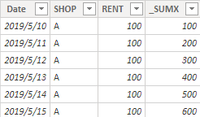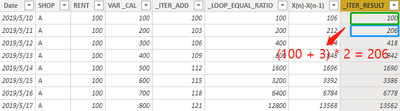cancel
Showing results for
Did you mean:## Iteration in DAX

In practical business, data iteration is widely used, such as present value or depreciation. However, as we all know, DAX is a functional language based on column engine. Each row of data is calculated based on its own row context. But sometimes what we need to achieve is iteration, that’s say, the data in row N is calculated based on the data in row N-1, this calculation can be easily achieved in Excel (such as A, B, C columns in the figure below)But in Power BI (or SSAS Tabular), although you can use functions like SUMX to realize the iteration operation as shown below,

``````_SUMX =
SUMX(
FILTER('TABLE',
'TABLE'[DATE]<=EARLIER('TABLE'[DATE])),
'TABLE'[RENT])
RETURN _SUMX``````or using CALCULATE() to get the same result,

``````VAR _CAL =
CALCULATE(
SUM('TABLE'[RENT]),
FILTER('TABLE',
'TABLE'[DATE]<=EARLIER('TABLE'[DATE])
)
)``````

it’s difficult to achieve the result of the second row to N row values when the column only has value on the first row. This calculation is very easy using an Excel function, but hard in DAX.

Next, I'll show you how to use DAX to solve these problems.

## Simple Iteration in DAX

DAX can also solve this problem perfectly. When you think about iteration in Power BI with sequence thinking, it will be much simpler. Therefore, for the case of column A in the above figure, when the N + 1 row of the column is equal to the nth row plus D, the data of the whole column is actually an arithmetic sequence with a tolerance of 2. As follows, we only need to use the formula of the arithmetic sequence:

``````_ITER_ADD =
VAR D = 3
VAR A1 = 100
VAR N =
CALCULATE(
COUNTROWS('TABLE'),
FILTER(
'TABLE',
'TABLE'[Date]<EARLIER('TABLE'[Date]))
)
RETURN
A1 + N * D    ``````In the same way, if the case is like column B:  X(n-1) = X(n) * 2, then this is an equal ratio sequence:

``````_LOOP_EQUAL_RATIO =
VAR Q = 2
VAR A1 = 100
VAR N =
CALCULATE(
COUNTROWS('TABLE'),
FILTER(
'TABLE',
'TABLE'[Date]<EARLIER('TABLE'[Date]))
)
RETURN
A1 * POWER(Q,N)    ``````Complex Iteration in DAX

As in column C of Figure 1. When the iterative formula class is like X(n + 1) = (X (n) + D) * Q, its logic is not as simple as the above formula, but in fact, this is a mathematical problem. The answer is: Although the sequence X(n) itself is an irregular sequence, X(n) - X(n-1) is a regular sequence, and it is an equal ratio sequence. According to this idea, we can first find X(n) - X(n-1) by using the rule of equal ratio sequence and then deduce X(n).

Firstly, the formula of X (n) - X (n-1) is as follows:

``````X(n)-X(n-1) =
VAR P_DATE = 'TABLE'[Date]
VAR D = 3
VAR Q = 2
VAR _RENT =
CALCULATE(
FIRSTNONBLANK('TABLE'[RENT],0),
'TABLE')
VAR _FIXED_DISTANCE = (_RENT+D)*Q - _RENT
VAR _LOOP_1 = GENERATESERIES(1,COUNTROWS('TABLE'))
VAR _LOOP_2 =
_FIXED_DISTANCE*PRODUCTX(
FILTER('TABLE','TABLE'[Date]<P_DATE),Q))
VAR _MAX = MAXX(_LOOP_2,_RENT)
VAR VARIABLE_DISTANCE =
IF(
ISBLANK(
MAXX(
FILTER(_LOOP_2,_RENT=_MAX),
[_FIXED_DISTANCE])),
_FIXED_DISTANCE,
MAXX(
FILTER(_LOOP_2,_RENT=_MAX),
[_FIXED_DISTANCE]))
RETURN
VARIABLE_DISTANCE``````After calculating the value of X(n) - X(n-1), we can deduce the value of X(n), because X(n) is a sequence with X(n) -X(n-1) as the tolerance.

``````_ITER_RESULT =
VAR P_DATE = 'TABLE'[DATE]
VAR _RENT =
CALCULATE(
FIRSTNOBLANK('TABLE'[RENT],0),
ALL('TABLE')
)
VAR _LOOP_1 = GENERATESERIES(1,COUNTROWS('TABLE'))
VAR _LOOP_2 =
_RENT+SUMX(FILTER('TABLE',
'TABLE'[DATE]<P_DATE),
'TABLE'[F(n)-F(n-1)])
)
VAR _MAX = MAXX(_LOOP_2,_RENT)
VAR _RESULT =
MAXX(FILTER(_LOOP_2,_RENT=_MAX),
[_RENT])
RETURN _RESULT``````In this way, we have completed the iterative calculation under this complex formula. After testing, no matter how we change the value of D and Q in the formula, the formula returns the correct iteration result perfectly!

Final Version

``````_RESULT =
VAR P_DATE = 'TABLE'[Date]
VAR D = 3
VAR Q = 2
VAR _RENT =
CALCULATE ( FIRSTNONBLANK ( 'TABLE'[RENT], 0 ), 'TABLE' )
VAR _FIXED_DISTANCE = ( _RENT + D ) * Q - _RENT
VAR _LOOP_1 =
GENERATESERIES ( 1, COUNTROWS ( 'TABLE' ) )
VAR _LOOP_2 =
_LOOP_1,
"_FIXED_DISTANCE", _FIXED_DISTANCE
* PRODUCTX ( FILTER ( 'TABLE', 'TABLE'[Date] < P_DATE ), Q )
)
VAR _MAX =
MAXX ( _LOOP_2, _RENT )
VAR VARIABLE_DISTANCE =
IF (
ISBLANK ( MAXX ( FILTER ( _LOOP_2, _RENT = _MAX ), [_FIXED_DISTANCE] ) ),
_FIXED_DISTANCE,
MAXX ( FILTER ( _LOOP_2, _RENT = _MAX ), [_FIXED_DISTANCE] )
) - D * 2
RETURN
VARIABLE_DISTANCE``````

## Consideration

Although DAX can solve this problem, for complex iterative calculation, I suggest that you use other methods, such as SQL or R, to pre-calculate the result set, and then load it to Power BI for display, this can greatly save memory load.